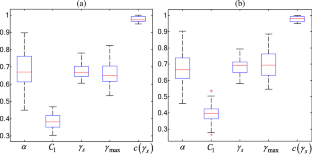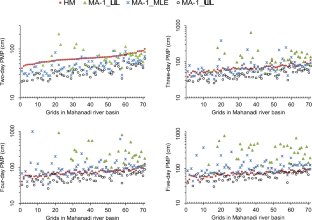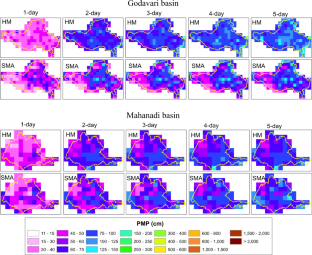# Evaluation of three approaches to probable maximum precipitation estimation: a study on two Indian river basins

## Abstract

Estimates of probable maximum precipitation (PMP) and corresponding probable maximum flood (PMF) are necessary for planning, design, and risk assessment of flood control structures whose failure could have catastrophic consequences. For PMP estimation, multifractal approach (MA) is deemed to be better than conventional approaches, which are based either on statistical concepts or physical aspects. The MA yields physically meaningful PMP estimates by attempting to capture scale-invariant multiplicative cascade mechanism inherent in rainfall. This paper attempts to gain insights into the performance of MA by comparing PMP estimates obtained using the approach with those resulting from the use of two widely used empirical approaches (storm maximization approach (SMA) and Hershfield method (HM)) on two flood-prone river basins (Mahanadi and Godavari) in India. The results indicate that rainfall data of the two river basins exhibit multifractal properties, and the use of MA has an advantage over HM and SMA in estimating PMP corresponding to longer durations (>3 days). PMP estimates obtained using HM are generally lower (higher) than those obtained using SMA for 1-day (higher) duration. PMP maps are prepared for the two Indian river basins corresponding to 1-day to 5-day durations. Further, PMP estimates obtained based on the PMP maps are provided for 18 catchments in the Mahanadi basin and 53 catchments in the Godavari river basin.

This is a preview of subscription content, access via your institution.Fig. 1Fig. 2Fig. 3Fig. 4Fig. 5Fig. 6Fig. 7Fig. 8Fig. 9Fig. 10Fig. 11Fig. 12Fig. 13Fig. 14Fig. 15

## Data availability

Daily gridded precipitation data having a resolution of 0.5°×0.5° was procured from the India Meteorological Department (IMD). Data is available for research purposes only.

## References

1. Arora M, Kumar R, Singh RD, Malhotra J, Kumar N (2016) Analysis of unusual meteorological conditions that led to recent floods in Bhagirathi Basin (Uttarakhand Himalayas). Hydrol Sci J 61(7):1238–1243

2. Bernardara P, Schertzer D, Sauquet E, Tchiguirinskaia I, Lang M (2008) The flood probability distribution tail: how heavy is it? Stoch Env Res Risk A 22(1):107–122

3. Central Water Commission (CWC) (2012) Integrated hydrological data book. Hydrological data directorate, information systems organization, water planning and projects wing, New Delhi, India

4. Chavan SR, Srinivas VV (2017) Regionalization based envelope curves for PMP estimation by Hershfield method. Int J Climatol 37(10):3767–3779

5. Chen LC, Bradley AA (2006) Adequacy of using surface humidity to estimate atmospheric moisture availability for probable maximum precipitation. Water Resour Res 42(9)

6. Chen CT, Knutson T (2008) On the verification and comparison of extreme rainfall indices from climate models. J Clim 21(7):1605–1621

7. Chow VT, David RM, Larry WM (1988) Applied hydrology. McGraw-Hill

8. Coles S, Bawa J, Trenner L, Dorazio P (2001) An introduction to statistical modeling of extreme values, vol 208. Springer, London

9. Collier CG, Hardaker PJ (1996) Estimating probable maximum precipitation using a storm model approach. J Hydrol 183:277–306

10. De Lima MIP, Grasman J (1999) Multifractal analysis of 15-min and daily rainfall from a semi-arid region in Portugal. J Hydrol 220(1):1–11

11. Deshpande NR, Kulkarni BD, Verma AK, Mandal BN (2008) Extreme rainfall analysis and estimation of Probable Maximum Precipitation (PMP) by statistical methods over the Indus river basin in India. J Spat Hydrol 8(1)

12. Dooge JC (1959) A general theory of the unit hydrograph. J Geophys Res 64(2):241–256

13. Dooge JCI (1986) Looking for hydrologic laws. Water Resour Res 22(9):46S–58S

14. Douglas EM, Barros AP (2003) Probable maximum precipitation estimation using multifractals: application in the Eastern United States. J Hydrometeorol 4:1012–1024

15. Gupta VK, Waymire E (1990) Multiscaling properties of spatial rainfall and river flow distributions. J Geophys Res 95(D3):1999–2009

16. Hershfield DM (1961) Estimating the probable maximum precipitation. J Hydraul Div Am Soc Civ Eng 87(HY5):99–106

17. Hershfield DM (1965) Method for estimating probable maximum rainfall. J Am Water Works Assoc 57:965–972

18. Hubert P, Tessier Y, Lovejoy S, Schertzer D, Schmitt F, Ladoy P, Carbonnel JP, Violette S, Desurosne I (1993) Multifractals and extreme rainfall events. Geophys Res Lett 20(10):931–934

19. Jain SK, Agarwal PK, Singh VP (2007) Hydrology and water resources of India (Vol. 57). Springer Science & Business Media.

20. Jarvis A, Reuter HI, Nelson A, Guevara E (2008) Hole-filled SRTM for the globe Version 4, available from the CGIAR-CSI SRTM 90m Database (http://srtm.csi.cgiar.org)

21. Kalnay E, Kanamitsu M, Kistler R, Collins W, Deaven D, Gandin L, Iredell M, Saha S, White G, Woollen J, Zhu Y, Chelliah M, Ebisuzaki W, Higgins W, Janowiak J, Mo KC, Ropelewski C, Wang J, Leetmaa A, Reynolds R, Jenne R, Joseph D (1996) The NCEP/NCAR 40-year reanalysis project. B Am Meteorol Soc 77(3):437–471

22. Koutsoyiannis D (1999) A probabilistic view of Hershfield’s method for estimating probable maximum precipitation. Water Resour Res 35:1313–1322

23. Kulkarni AK, Mandal BN, Sangam RB (1998) Estimation of probable maximum precipitation (PMP) of the Sina river catchment in Maharashtra for design purposes. Maharashtra Bhugol Shastra Sansthan Patrika 12:135–148

24. Lavallée D, Schertzer D, Lovejoy S (1991) On the determination of the codimension function. In: Non-linear variability in geophysics. Springer, Netherlands, pp 99–109

25. London J (1962) A study of the atmospheric heat balance. Research Division, College of Engineering, Department of Meteorology and Oceanography, New York University

26. Lovejoy S, Schertzer D (1985) Generalized scale invariance in the atmosphere and fractal models of rain. Water Resour Res 21(8):1233–1250

27. Lovejoy S, Schertzer D (1990) Multifractals, universality classes and satellite and radar measurements of cloud and rain fields. J Geophys Res-Atmos 95(D3):2021–2034

28. Lovejoy S, Schertzer D (2007) Multifractals and rain. In: Kundzewicz ZW (ed) New uncertainty concepts in hydrology and water resources. Cambridge University Press, Cambridge, pp 61–103

29. Lu F, Wang H, Yan D, Zhang D, Xiao W (2013) Application of profile likelihood function to the uncertainty analysis of hydrometeorological extreme inference. Sci China Technol Sci 56(12):3151–3160

30. Olsson J (1995) Limits and characteristics of the multifractal behavior of a high-resolution rainfall time series. Nonlinear Process Geophys 2:23–29

31. Osborn TJ, Hulme M (1997) Development of a relationship between station and grid-box rainday frequencies for climate model evaluation. J Clim 10(8):1885–1908

32. Papalexiou SM, Koutsoyiannis D (2006) A probabilistic approach to the concept of Probable Maximum Precipitation. Adv Geosci 7:51–54

33. Papalexiou SM, Koutsoyiannis D (2013) Battle of extreme value distributions: a global survey on extreme daily rainfall. Water Resour Res 49(1):187–201

34. Parisi G, Frisch U (1985) A multifractal model of intermittency. In: Ghil M, Benzi R, Parisi G (eds) Turbulence and predictability in geophysical fluid dynamics and climate dynamics. Elsevier, North-Holland, pp 84–88

35. Rajeevan M, Bhate J (2008) A high resolution daily gridded rainfall data set (1971-2005) for Mesoscale Meteorological Studies. NCC research report no.9

36. Rajeevan M, Bhate J (2009) A high resolution daily gridded rainfall dataset (1971-2005) for mesoscale meteorological studies. Curr Sci 96(4):558–562

37. Rakhecha PR, Clark C (2002) The probable maximum flood at the Ukai and Lakhwar dam sites in India. In: The extremes of lite extremes: Extraordinary Floods, July 2000, Reykjavik, Iceland

38. Rakhecha PR, Soman MK (1994) Estimation of probable maximum precipitation for a 2-day duration: part 2—North Indian region. Theor Appl Climatol 49(2):77–84

39. Rakhecha PR, Deshpande NR, Soman MK (1992) Probable maximum precipitation for a 2-day duration over the Indian peninsula. Theor Appl Climatol 45:277–283

40. Rakhecha PR, Mandal BN, Kulkarni AK, Deshpande NR (1995) Estimation of probable maximum precipitation for catchments in eastern India by a generalized method. Theor Appl Climatol 51(1-2):67–74

41. Rakhecha PR, Kulkarni AK, Mandal BN, Sangam RB, Deshpande NR (1998) Probable maximum precipitation for the catchment of Koyna dam. Mausam 49:211–216

42. Rao AR, Hamed KH (2000) Flood Frequency Analysis. CRC Press, Boca Raton

43. Schertzer D, Lovejoy S (1987) Physical modeling and analysis of rain and clouds by anisotropic scaling of multiplicative processes. J Geophys Res 92D:9693–9714

44. Schertzer D, Lovejoy S (1992) Hard and soft multifractal processes. Phys A 185(1):187–194

45. Smith WL (1966) Note on the relationship between total precipitable water and surface dew point. J Appl Meteorol 5(5):726–727

46. Srivastava AK, Rajeevan M, Kshirsagar SR (2009) Development of a high resolution daily gridded temperature data set (1969-2005) for the Indian region. Atmos Sci Lett. https://doi.org/10.1002/asl.232

47. Swain RE, England JF, Bullard KL, Raff DA (2004) Hydrologic hazard curve estimating procedures. Research report DSO-04-08, U.S. Department of Interior, Bureau of Reclamation

48. Tessier Y, Lovejoy S, Schertzer D (1993) Universal multifractals: theory and observations for rain and clouds. J Appl Meteorol 32:223–250

49. Tessier Y, Lovejoy S, Hubert P, Schertzer D, Pecknold S (1996) Multifractal analysis and modeling of rainfall and river flows and scaling, causal transfer functions. J Geophys Res-Atmos 101(D21):26427–26440

50. Volosciuk C, Maraun D, Semenov VA, Park W (2015) Extreme precipitation in an atmosphere general circulation model: impact of horizontal and vertical model resolutions. J Clim 28(3):1184–1205

51. World Meteorological Organization (WMO) (1986) Manual for estimation of probable maximum precipitation, Operational Hydrology Report No. 1, 2nd edition, WMO No. 332, Secretariat of the World Meteorological Organization, Geneva, Switzerland

52. World Meteorological Organization (WMO) (2009) Manual on estimation of Probable Maximum Precipitation (PMP), World Meteorological Organization, WMO-No. 1045, Geneva, Switzerland

Download references

## Acknowledgements

The authors express their gratitude to the Editor for the constructive reviews that resulted in improving quality of the work. The authors are also thankful to the India Meteorological Department, which provided the gridded rainfall data for the study.

Not applicable.

## Author information

Authors

### Contributions

Sagar Rohidas Chavan: formal analysis, investigation, writing-original draft. V. V. Srinivas: investigation, writing- review and editing.

### Corresponding author

Correspondence to Sagar Rohidas Chavan.

## Ethics declarations

### Conflict of interest

The authors declare no competing interests.

## Additional information

### Publisher’s note

Springer Nature remains neutral with regard to jurisdictional claims in published maps and institutional affiliations.

(DOCX 1216 kb)

## Appendixes

### Generalized extreme value distribution

The GEV distribution is a continuous probability distribution which is useful to model annual maximum values x1, …, xm extracted from the observed precipitation data. The cumulative distribution function F of the GEV distribution is of the form:

$$F(x)=\left\{\begin{array}{l}\exp \left\{-{\left[1+k\frac{\left(x-\xi \right)}{\beta}\right]}^{-1/k}\right\}\kern0.5em \mathrm{for}\ k\ne 0\\ {}\exp \left\{-\exp \left[\frac{\left(x-\xi \right)}{\beta}\right]\right\}\kern2em \mathrm{for}\ k=0\end{array}\right.$$
(A1)

where ξ, β, and k are the location, scale, and shape parameters respectively (β > 0, ξ, k ∈ ) and 1 + k(x − ξ)/β > 0. Estimates of the parameters are obtained in this study using the maximum likelihood method. Equation (A1) can be rearranged to get $${\hat{x}}_F$$ (i.e., the quantile estimate of x) for any specified value of non-exceedance probability F (where 0 < F < 1).

$${\hat{x}}_F=\left\{\begin{array}{l}\hat{\xi}-\frac{\hat{\beta}}{\hat{k}}\left(1-{\left(-\log F\right)}^{-\hat{k}}\right),\kern0.75em \hat{k}\ne 0\\ {}\hat{\xi}-\hat{\beta}\log \left(-\log F\right)\kern2.5em \hat{k}=0\end{array}\right.$$
(A2)

The shape parameter k is important, as it determines the tail behavior of the distribution. If k > 0, it will correspond to Fréchet distribution, which has a long tail. If k = 0, it is referred to as Gumbel distribution whose tail is exponential. If k < 0, it will correspond to Weibull distribution with an upper limit for x. The sampling error caused by the limited sample data (observations on the extreme precipitation) is inevitable, which increases the uncertainty in $$\hat{k}$$ and consequently xF that depends on $$\hat{k}$$. The uncertainty can be assessed by constructing the profile likelihood function to determine the confidence intervals of the shape parameter k and the quantile estimate xF (e.g., Coles et al. 2001; Lu et al. 2013).

For a given value of precipitation (PMP), the corresponding return period T can be estimated in the case of GEV distribution as,

$$T=\frac{1}{1-\exp \left\{-{\left[1+\frac{k}{\beta}\left(\mathrm{PMP}-\xi \right)\right]}^{-1/k}\right\}}$$
(A3)

### Concept of profile likelihood function

In analysis with GEV distribution, the procedure to estimate confidence intervals of the shape parameter k and the quantile estimate xF using profile likelihood function (Coles et al. 2001; Lu et al. 2013) involves the following steps.

1. (1)

Determine the maximum likelihood estimates of parameters $$\left(\hat{\xi},\hat{\beta},\hat{k}\right)$$ and quantile xF of the GEV distribution, where $$\hat{\xi}$$ and $$\hat{\beta}$$ denote location and scale parameters, respectively.

Let x1, …, xm denote the data points in the annual maximum precipitation record. Construct the log-likelihood function to estimate parameters of GEV distribution. The function is given by Equation (A4) when k ≠ 0, and by Equation (A5) if k = 0.

$$\mathrm{\ell}\left(\xi, \beta, k\right)=-m\log \beta -\left(1+1/k\right)\times \sum \limits_{i=1}^m\log \left[1+k\left(\frac{x_i-\xi }{\beta}\right)\right]-\sum \limits_{i=1}^m{\left[1+k\left(\frac{x_i-\xi }{\beta}\right)\right]}^{-1/k}$$
(A4)
$$\mathrm{\ell}\left(\mu, \beta \right)=-m\log \beta -\sum \limits_{i=1}^m\left(\frac{x_i-\xi }{\beta}\right)-\sum \limits_{i=1}^m\exp \left\{-\left(\frac{x_i-\xi }{\beta}\right)\right\}$$
(A5)

Maximize the log-likelihood function to determine the maximum likelihood estimates of the parameters $$\left(\hat{\xi},\hat{\beta},\hat{k}\right)$$. Use the estimated values of the parameters in Equation (A2) to arrive at a quantile estimate xF corresponding to non-exceedance probability F (where 0 < F < 1).

1. (2)

Determine profile likelihood estimate of the shape parameter k of GEV distribution.

Suppose the shape parameter k = k0 ≠ 0 is a constant. Maximize the log-likelihood function given by Equation (A4) about ξ and β, repetitively in a certain range of k0. For each value assumed for k0, the maximum value of the likelihood function can be obtained. The profile likelihood function of k is constructed using the resulting information. Finally, an approximation of 1 − α confidence interval of k is determined by using $${C}_{\alpha }=\left\{k:2\left(\mathrm{\ell}\left(\hat{\xi},\hat{\beta},\hat{k}\right)-\underset{\xi, \alpha }{\max}\mathrm{\ell}\left(\xi, \beta, k\right)\right)\le {c}_{1-\alpha}\right\}$$, where c1 − α is 1 − α quantile of chi-square distribution with one degree of freedom.

1. (3)

Determine profile likelihood estimate of quantile xF of GEV distribution

To obtain a profile likelihood estimate of annual maximum precipitation quantile xF, redefine the parameters of GEV distribution by introducing xF into the likelihood function. It can be achieved by rearranging Equation (A2) to get Equation (A6) and substituting Equation (A6) into Equation (A4) to derive the log-likelihood function of GEV as a function of (xF, β, k).

$$\xi =\left\{\begin{array}{l}{x}_F+\frac{\beta }{k}\left(1-{\left(-\log F\right)}^{-k}\right),\kern0.75em k\ne 0\\ {}{x}_F+\beta \log \left(-\log F\right)\kern2.5em k=0\end{array}\right.$$
(A6)

Finally, the profile likelihood estimate and confidence interval of quantile xF of GEV distribution can be obtained by following the procedure described (for shape parameter k) in step (2).

### Area weighted PMP for a catchment

To estimate PMP for the catchment of a stream gauge in a river basin, the following steps were executed.

1. (1)

Catchment of the gauge was delineated by processing SRTM DEM data using ArcHYDRO tools in the ArcGIS framework.

2. (2)

Area weighted PMP corresponding to 1-day to 5-day durations was estimated for the catchment based on PMP map prepared for the basin as,

$${\mathrm{PMP}}^{(c)}=\sum \limits_{i=1}^{N_c}{w}_i\times {\mathrm{PMP}}_i=\sum \limits_{i=1}^{N_c}\left(\frac{A_i^{(c)}}{CA}\right)\times {\mathrm{PMP}}_i$$
(A7)

where PMP(c) denotes area-weighted PMP for a catchment c having area CA and comprising Nc grids, $${A}_i^{(c)}$$ denotes the area of i-th grid (i = 1, …, Nc) contained in the catchment, and wi is the weight assigned to PMPi (i.e., PMP corresponding to i-th grid) as the ratio of $${A}_i^{(c)}$$ to CA.

## Rights and permissions

Reprints and Permissions